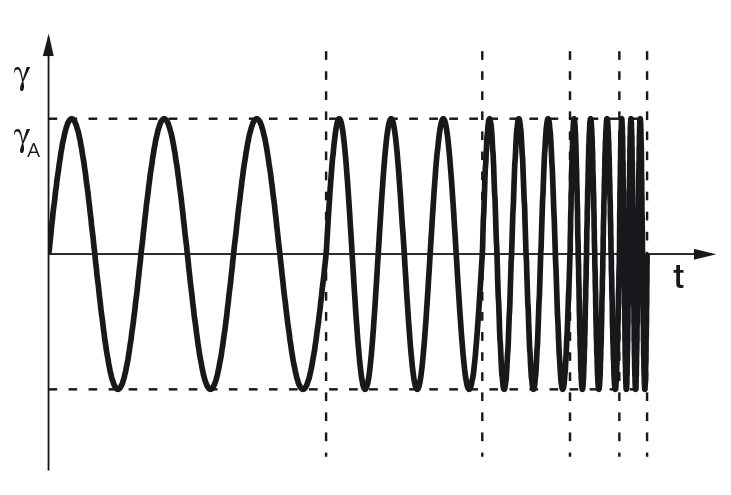# Frequency sweepsFigure 1: Preset of a frequency sweep, here with controlled shear strain and an increase or decrease in frequency in five steps. The strain amplitude γA is kept constant over all five measuring points.

Frequency sweeps generally serve the purpose of describing the time-dependent behavior of a sample in the non-destructive deformation range. High frequencies are used to simulate fast motion on short timescales, whereas low frequencies simulate slow motion on long timescales or at rest. In practice, frequency sweeps are proven methods for gathering information on the behavior and inner structure of polymers as well as on the long-term stability of dispersions.

Many users prefer the method of measuring from maximum to minimum frequency as this often results in a shorter testing period due to a shorter adjustment time of the controller. The same result is obtained for either method if, on the one hand, the measurement takes place within the LVE region and if, on the other hand, the sample shows no change during the test period. There are two modes of operation for presetting the deflection:

a) Controlled strain (or controlled shear deformation CSD)
b) Controlled shear stress (CSS)

The precondition is that the selected shear-strain or shear-stress amplitude is within the LVE region. This means that the limit of the LVE region must first be determined in an amplitude sweep. As explained previously for amplitude sweeps, frequencies can be stated in one of two ways: As frequency f in Hz or as angular frequency ω in rad/s or in s-1. It is advisable to work with angular frequencies because there are SI units available. To convert between the two frequencies, the following holds, with circle constant π = 3.14: ω (in rad/s) = 2π ⋅ f (in Hz).

The results of frequency sweeps are usually presented in a diagram with the (angular) frequency plotted on the x-axis and storage modulus G' and loss modulus G'' plotted on the y-axis, with both axes on a logarithmic scale (Figure 1).